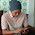# Addition and subtraction method of a complex numbers

Addition and subtraction method of a complex numbers given by the problem, solved in rectangular form. Where has the rule: real part added (or subtracted) with real part, and imaginary part added (or subtracted) with imaginary part of a complex numbers.

Sample question 1: (30 + j25) + (13 − j5) = ?

Completion:

x (real) = 30 + 13 = 43

y (imaginary) = 25 − 5 = 20

Result:

(30 + j25) + (13 − j5) = 43 + j20

If there is a question addition (or subtraction) of polar and rectangular, then the polar form must be converted into rectangular form.

Sample question 2: (53 + j17) + (21 ∠ 22°) = ?

Completion:

Conversion polar form (21 ∠ 22°) into rectangular form

21 ∠ 22° = 21 (cos 22 + jsin 22) = 19.47086 + j7.86674

we have

x (real) = 53 + 19.47086 = 72.47086

y (imaginary) = 17 + 7.86674 = 24.86674

Result:

(53 + j17) + (21 ∠ 22°) = 72.47086 + j24.86674
Next→
←Prev

### Choose label

#### 1 comment

1.بِسْمِ اللهِ الرَّحْمٰنِ الرَّحِيْمِ

اَلْحَمْدُ ِللهِ رَبِّ الْعٰلَمِيْنَ

اَلرَّحْمٰنِ الرَّحِيْمِ

سُكْرًا

:senyum: